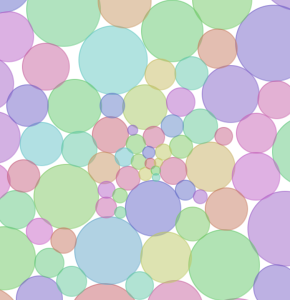# Matthias Shapiro

I need fewer hobbies

# Circle Packing Algorithm in Processing

This is based on this Processing sketch on circle packing, but since I had to update it to get it to work, I figured I’d just post my alterations on the code.

In case you’re not familiar with circle packing, it is an iterative algorithm for finding the lowest stable state of a set of 2D circles.// Part 1: Set up, draw, compare

import java.util.Comparator;
import java.util.Arrays;

ArrayList circles;
long iterationCounter = 0;

void setup() {
size(1000, 1000 );
smooth();
fill( 0 );
frameRate( 20 );
circles = createRandomCircles(100);
background(255);
}

void draw() {
background( 255 );
for (int i=0; i<circles.size(); i++) {
getCircle(i).draw();
}
for (int i=1; i<50; i++) {
iterateLayout(i);
}
}
Comparator comp = new Comparator() {
public int compare(Object p1, Object p2) {
Circle a = (Circle)p1;
Circle b = (Circle)p2;
if (a.distanceToCenter() < b.distanceToCenter())
return 1;
else if (a.distanceToCenter() > b.distanceToCenter())
return -1;
else
return 0;
}
};

// Part 2: Iterate the layout

void iterateLayout(int iterationCounter) {
Object circs[] = circles.toArray();
Arrays.sort(circs, comp);

//fix overlaps
Circle ci, cj;
PVector v = new PVector();
for (int i=0; i<circs.length; i++) {
ci = (Circle)circs[i];
for (int j=i+1; j<circs.length; j++) {
if (i != j) {
cj = (Circle)circs[j];
float dx = cj.x – ci.x;
float dy = cj.y – ci.y;
float d = (dx*dx) + (dy*dy);
if (d < (r * r) – 0.01 ) {
v.x = dx;
v.y = dy;
v.normalize();
v.mult((r-sqrt(d))*0.5);
if (cj != dragCircle) {
cj.x += v.x;
cj.y += v.y;
}

if (ci != dragCircle) {
ci.x -= v.x;
ci.y -= v.y;
}
}
}
}
}

//Contract
float damping = 0.1/(float)(iterationCounter);
for (int i=0; i<circs.length; i++) {
Circle c = (Circle)circs[i];
if (c != dragCircle) {
v.x = c.x-width/2;
v.y = c.y-height/2;
v.mult(damping);
c.x -= v.x;
c.y -= v.y;
}
}
}

// Part 3: Create initial circles, handle interactions

ArrayList createRandomCircles(int n) {
ArrayList circles = new ArrayList();
colorMode(HSB, 255);
while (n– > 0) {
Circle c = new Circle(random(width), random(height), (n)+10);
c.myColor = color(random(255), 128, 200, 128);
}
colorMode(RGB,255);
return circles;
}

Circle getCircle(int i) {
return (Circle)circles.get(i);
}

Circle dragCircle = null;

void keyPressed() {
circles = createRandomCircles(50);
}

void mousePressed() {
dragCircle = null;
for(int i=0; i<circles.size(); i++) {
Circle c = getCircle(i);
if (c.contains(mouseX, mouseY)) {
dragCircle = c;
}
}
}

void mouseDragged() {
if (dragCircle != null) {
dragCircle.x = mouseX;
dragCircle.y = mouseY;
}
}

void mouseReleased() {
dragCircle = null;
}

// Part 4: The Circle class
class Circle {
public color myColor;

public Circle(float x, float y, float radius) {
this.x = x;
this.y = y;
myColor = color(64,64,64,64);
}

public void draw() {
fill(myColor);
stroke(myColor);
strokeWeight(3);
}

public boolean contains(float x, float y) {
float dx = this.x – x;
float dy = this.y – y;
return sqrt(dx*dx + dy*dy) <= radius;
}

public float distanceToCenter() {
float dx = x – WIDTH/2;
float dy = y – HEIGHT/2;
return (sqrt(dx*dx + dy*dy));
}

public boolean intersects(Circle c) {
float dx = c.x – x;
float dy = c.y – y;
float d = sqrt(dx*dx + dy*dy);